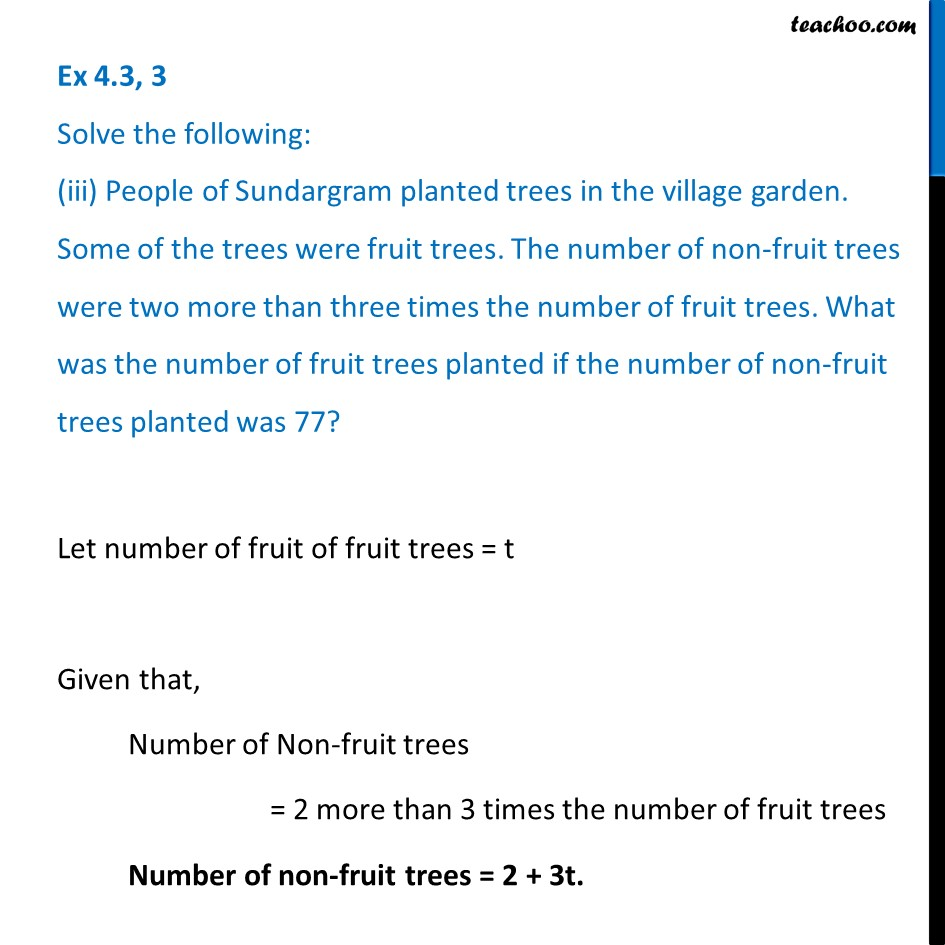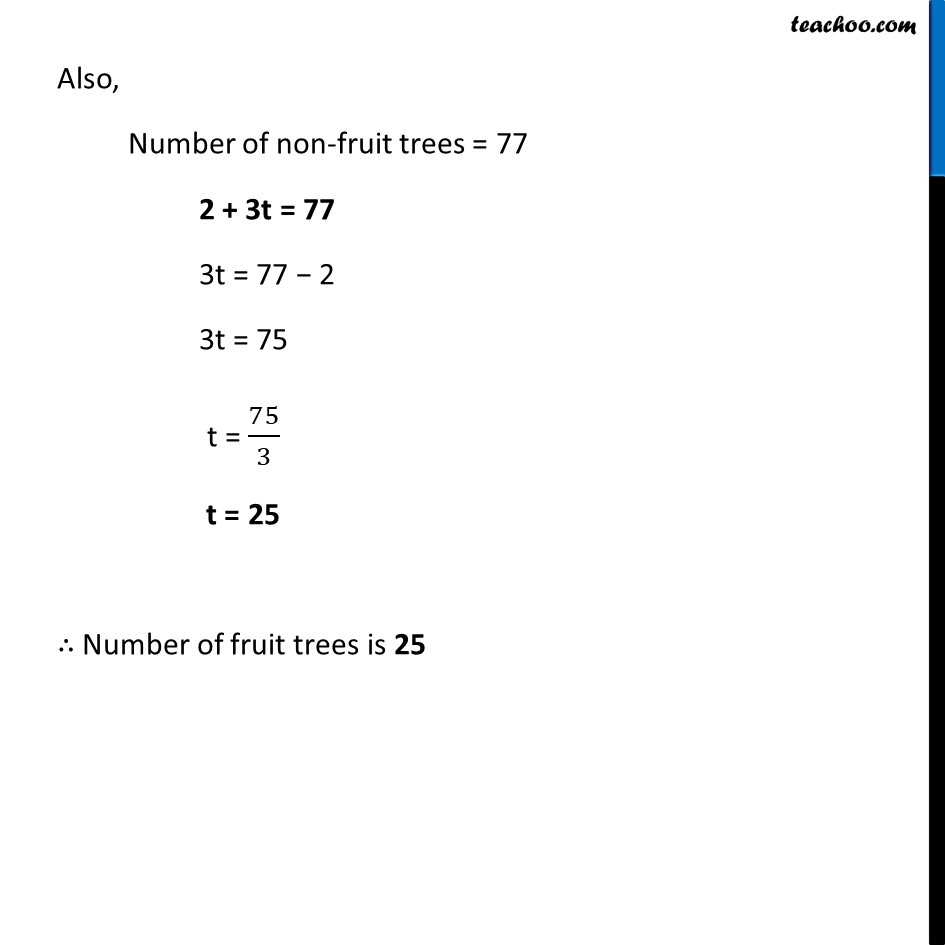Ex 4.3

Chapter 4 Class 7 Simple Equations
Serial order wiseLearn in your speed, with individual attention - Teachoo Maths 1-on-1 Class

### Transcript

Ex 4.3, 3 Solve the following: (iii) People of Sundargram planted trees in the village garden. Some of the trees were fruit trees. The number of non-fruit trees were two more than three times the number of fruit trees. What was the number of fruit trees planted if the number of non-fruit trees planted was 77?Let number of fruit of fruit trees = t Given that, Number of Non-fruit trees = 2 more than 3 times the number of fruit trees Number of non-fruit trees = 2 + 3t. Also, Number of non-fruit trees = 77 2 + 3t = 77 3t = 77 − 2 3t = 75 t = 75/3 t = 25 ∴ Number of fruit trees is 25